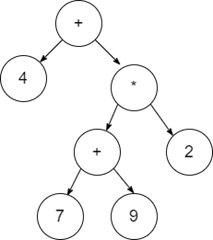# Algorithm to construct an Expression Tree in Data Structure

## Expression trees

Expression trees are those in which the leaf nodes have the values to be operated, and internal nodes contain the operator on which the leaf node will be performed.

## Example

4 + ((7 + 9) * 2) will have an expression tree as follows## Algorithm to Construct an Expression Tree

Let T be the expression tree.

If T is not NULL:   If T->data is an operand:      return T.data   A = solve(T.left)   B = solve(T.right)   --> Calculate operator for 'T.data' on A and B, and call recursively,      return calculate(A, B, T.data)

## How to construct an expression tree?

To construct an Expression Tree for the given expression, we generally use Stack Data Structure.

Initially we Iterate over the given postfix expression and follow the steps as given below -

• If we get an operand in the given expression, then push it in the stack. It will become the root of the expression Tree.
• If an operator gets two values in the expression, then add in the expression tree as its child, and push them in the current node.
• Repeat Step-1 and Step-2 until we do not complete over the given expression.
• Now check if every root node contains nothing but operands and every child node contains only values.